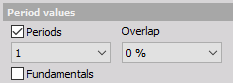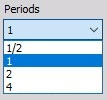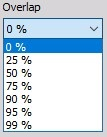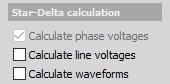# Power

## Basic calculationsBasic calculations are always calculated and displayed in Channel list.

## Period valuesWe can calculate Period values = values for voltage, current and power for:

• Periods = each = 1, 1/2, 2 or 4 period can be selected from drop down list• Overlap = 0%, 25%, 50% or 75%, 90%, 95%, 99% which can be selected from drop down listThis is very helpful for triggering.

## Star-Delta calculation

• To calculate line voltage check Calculate line voltage option.
• If we measure line to ground voltages, we can calculate Waveforms for the line to line voltages by selecting Calculate waveforms option.